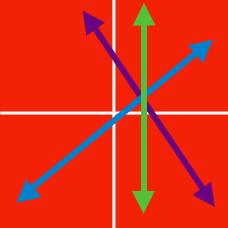Algebra

# System of Linear Equations - More Variables

Consider a system of linear equations \begin{aligned} x+y &= 3, \\ y+z &= 11, \\ z+x &= 20. \end{aligned} What is the value of $3x+y+5z$?

Let $x=a$, $y=b$ and $z=c$ be the values of $x$, $y$ and $z$, respectively, that satisfy the system of linear equations \begin{aligned} x-y&=3 \\ y+z&=25 \\ z-x&=8. \end{aligned} What is the value of $a+b+c$?

Let $x=a$, $y=b$ and $z=c$ be the values of $x$, $y$ and $z$, respectively, that satisfy the system of linear equations \begin{aligned} x+2y&= 24 \\ 2y+3z&= 58\\ x+3z&= 38. \end{aligned} What is the value of $a+b+c$?

Let $x=a$, $y=b$ and $z=c$ be the values of $x$, $y$ and $z$, respectively, that satisfy the system of linear equations $\frac{3-x}{2}= \frac{y+1}{3}=\frac{2z-1}{5}, \, 3x+2y+z=65.$ What is the value of $a+b+c$?

Let $x=A$, $y=B$ and $z=C$ be the solutions of the simultaneous equations \begin{aligned} x+y+z &= 270 \\ x-y+z &= 90 \\ x+y-z &=0. \end{aligned} What is the value of $\frac{A+B}{C}$?

×